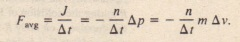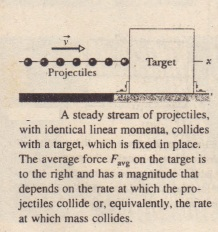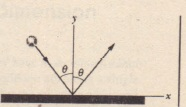# Series of Collisions

Series of Collisions

Now let’s consider the force on a body when it undergoes a series of identical, repeated collisions. For example, as a prank, we might adjust one of those machines that fires tennis balls to fire them at a rapid rate directly at a wall. Each collision would produce a force on the wall, but that is not the force we are seeking. We want the average force on the wall during the bombardment-that is, the average force during a large number of collisions.

By rearranging and substituting, we find the average force acting on the target during the collisions.This equation gives the average force  in terms of the rate at which mass collides with the target. Here again we can substitute for  depending on what the projectiles do.

CHECKPOINT 2: The figure shows an overhead view of a ball bouncing from a vertical wall without any change in its speed. Consider the change in the ball’s linear momentum. (a) Is  positive, negative, ? (b) Is , positive, negative, or zero? (c) What is the direction of .